# How to Calculate CAGR in Excel (Compound Annual Growth Rate Formula)

Posted May 27, 2019 by Sheraz Ali in Microsoft ExcelTo calculate CAGR in Excel, there’s no preset Excel function. However, there’s an easy method with which you can input a custom Excel formula and calculate it.

## So What is CAGR or Compound Anual Growth Rate?

If the annual growth rate of your company fluctuates over a period, CAGR is needed. It tells you the constant rate at which the sales of your company would have increased had it not fluctuated to meet its final fiscal year target.

## How to Calculate CAGR in Excel: An Example

If the concept of CAGR is still not clear to you, consider the following example.

1. The growth of a supposed company from the end of 2013 to the end of 2017 is given below. As you can see the growth never remained consistent. It changed from 16% to 34% to 21.30% to 8.40%.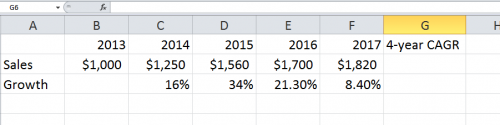We want to calculate a steady and consistent annual growth rate.

The formula you will input in excel is as follows.

2. Formula to Calculate CAGR in Excel

= (Cell of Last Year – Cell of First Year) ^ ( 1/No. of years)-1

3. So, in the following example, the cell of the first and last years is F2 and B2 respectively. And the number of years is 4. We input our values.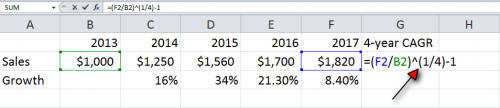• Note: Even though there are 5 years listed, we will only consider 4 while calculating CAGR because it is calculated from the end of the first year to the end of the last year and not from the start.

4. Press Enter to get the CAGR.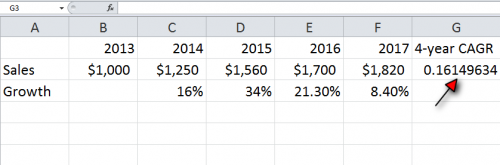5. To get the percentage CAGR, we have to format the cells to apply the percentage function. To do this, right-click the cell reference G and choose format cells from the drop-down menu.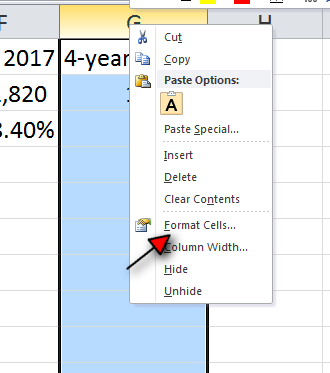6.  Choose Percentage and the number of decimal places you want to round off your answer to and Press OK to get %CAGR.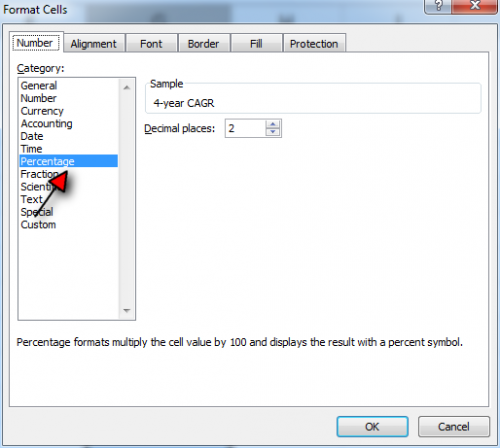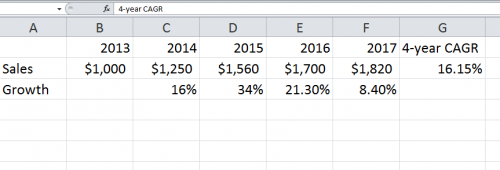That’s it! This is how easy it is to calculate CAGR in excel! No complicated formulae or sophisticated methodologies required!

If you’re interested in similar easy-to-follow, step-by-step Excel tutorials, check out the articles below. They will work wonders to hone your knowledge of Excel.

Calculate Standard Deviation in Excel

Freeze Panes in Excel

### Is there a function we can use to calculate CAGR in excel?

No, there’s no such function. You have to input the formula manually!

### What if I want to calculate the monthly growth rate instead of the CAGR.

Simple! You just substitute the number of years with the months and subtract the sales at the end of the last month from the end of the first month.

### What is the difference between IRR and CAGR in excel?

While you can calculate the IRR in excel with a function, it’s used for more complex operations than the CAGR. In essence, CAGR and IRR are the same but IRR deals with in-depth measurements, such as investment performance over a said period. CAGR, on the other hand, doesn’t account for investment performance.